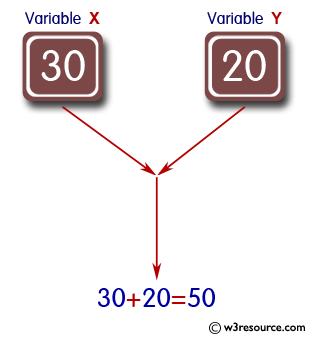﻿ Python: Print "30+20=50" - w3resource# Python: Print "30+20=50"

## Python Basic: Exercise-88 with Solution

Given variables x=30 and y=20, write a Python program to print "30+20=50".

Pictorial Presentation:Sample Solution-1:

Python Code:

``````x = 30
y = 20
print("\n%d+%d=%d" % (x, y, x+y))
print()
```
```

Sample Output:

```30+20=50
```

## Visualize Python code execution:

The following tool visualize what the computer is doing step-by-step as it executes the said program:

Sample Solution-2:

Python Code:

``````x = 30
y = 20
print("{0}+{1}={2}".format(x, y, x+y))
```
```

Sample Output:

```30+20=50
```

Sample Solution-3:

Python Code:

``````x = 30
y = 20
print("{}+{}={}".format(x, y, x + y))
```
```

Sample Output:

```30+20=50
```

Python Code Editor:

Have another way to solve this solution? Contribute your code (and comments) through Disqus.

What is the difficulty level of this exercise?

Test your Programming skills with w3resource's quiz.

﻿

## Python: Tips of the Day

Join Strings in an Iterable:

```>>> words = ('Hello', 'Python', 'Programmers')
>>> '!'.join(words)
'Hello!Python!Programmers'
>>> words_dict = {0: 'zero', 1: 'one', 2: 'two', 3: 'three'}
>>> '&'.join(words_dict.values())
'zero&one&two&three'
```